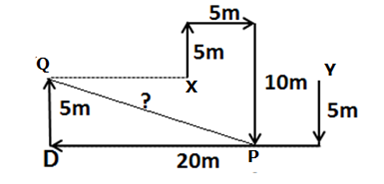# IBPS Clerk Mains Reasoning Questions 2019 – (Day-6)

Dear Aspirants, Our IBPS Guide team is providing new pattern Reasoning Questions for IBPS Clerk Mains 2019 so the aspirants can practice it on a daily basis. These questions are framed by our skilled experts after understanding your needs thoroughly. Aspirants can practice these new series questions daily to familiarize with the exact exam pattern and make your preparation effective.

“Ensure Your Ability Before of Exam “

## Take IBPS Clerk Free Mock Test 2019 – Start Now

[WpProQuiz 7421]

Seating arrangement

Directions (1-3): Study the following information to answer the given questions:

Six persons M, L, I, J, Q and S are sitting in a triangular table, Such that three of them sitting at corners of the table and three are sitting at middle of the each side. The persons who are sitting at corners are facing outside the centre and the person who sitting at middle of the sides are facing centre of the table. They like different Cricket players namely Dhoni, Sachin, Kholi, Raina, Rohit and Yuvi.

Q likes Kholi and sits second to right of the one who like Yuvi. J doesn’t faces opposite to the center. Only I sit immediate left of the one who like Sachin. L and J are not immediate neighbours. The one who like Rohit sits Second to the right of L. S doesn’t like Sachin. Two people sit between the one who like Sachin and the one who like Raina. Two People sits between M and the one who like Dhoni. The one who likes Raina sits second to the right of the one who like Dhoni.

1) Who sits second to the right of the one who like Yuvi?

a) The one who like Sachin

b) The one who sits second to the left of J

c) The one who like Raina

d) J

e) None of these

2) What is the position of J with respect to the one who like Raina?

a) Third to the right

b) Immediate left

c) Second to the left

d) Second to the right

e) Immediate right

3) Which of the following group of people faces the centre of the table?

a) I, S, Q

b) S, J, M

c) L, M, I

d) None of these

e) M, J, Q

Numerical Series

Directions (4-8): Study the following information to answer the given questions

There are two rows given. To find out the resultant of a particular row we need to follow the following steps: –

Step 1: If an even number is followed by an odd number (prime number) then the resultant will be the addition of both the numbers.

Step 2: If an odd number is followed by a perfect cube then the resultant will be the difference between the cube number and the odd number.

Step 3: If an odd number is followed by another odd number (but not a perfect square) then the resultant will be the addition of both the numbers.

Step 4: If an even number is followed by an odd (not a prime number) number then the resultant will be the difference between the odd number and the even number.

Step 5: If an odd number is followed by an even number (not a cube number) then the resultant will be multiplying the numbers.

4) If the sum of the resultants of two rows is 120. Then find the value of X?

6    21     X

7   11    25

a) 8

b) 7

c) 6

d) 9

e) None of the above

5) Find the sum of resultant of the first row and the second row?

3   6    9

4      7    27

a) 29

b) 31

c) 80

d) 25

e) None of the above

6) If X is double the value of resultant of the first row then what will be the resultant of the second row?

4     7    4

6     7     X

a) 484

b) 2144

c) 1444

d) 1144

e) None of these

7)  Which of the following is the resultant of second row?

3      7     35

24   31   64

a) 81

b) 59

c) 9

d) 82

e) None of these

8) Which of the following is the difference between the resultant of first row and the second row?

6   17   4

7    7   11

a) 40

b) 57

c) 15

d) None of these

e) 67

Data Sufficiency

Directions (9-10): Each of the questions below has question and followed by two statements I and II. You have to decide whether the data provided in the statements are sufficient to answer the question. Read the question and both the statements and give answer.

9) Eight persons – P, Q, R, S, T, U, V and W are belongs to same family but three different generations. How U is related to W?

Statements:

I) S is Mother of U. S married V who is son-in law of P. T is daughter in law of P who married Q. R is father of W and married T.

II) T is Son of U who has only one daughter. T married P. V is sister-in-law of P. V married S and has a daughter of W. S is son –in –law of Q who married U.

a) The data in statement I alone are sufficient to answer the question, while the data in statement II alone are not sufficient to answer the question.

b) The data in statement II alone are sufficient to answer the question, while the data in statement I alone are not sufficient to answer the question.

c) The data in either in statement I alone or statement II alone are sufficient to answer the question.

d) The data in both statements I and II are not sufficient to answer the question.

e) The data in both statements I and II are sufficient to answer the question.

10) Two person M and N starts walk from two different point X and Y respectively. What is the distance between two people after stopping at final point P and Q respectively?

Statements:

I) M walks 8m in north from point X , then he take right turn and walk 9m to reach point S and then he take left turn and stop at point P after walking 10m. N walks 18m in north direction from point Y which is 35m east from point X and the he turn left and walk 10m and then turn right and walk 5m to reach point A. Then he walks 5m in west direction and then walks 5m in south direction and stop at point Q.

II) M walks 5m in north direction from point X and take right and walks 5m, then walks 10m in south direction and stop at point P. N walks 5m in south direction from Point Y and turn right and walk 20m to reach point D and then he walks 5m in north direction and stop at point Q. Point X is exactly between point Q and Y.

a) The data in statement I alone are sufficient to answer the question, while the data in statement II alone are not sufficient to answer the question.

b) The data in statement II alone are sufficient to answer the question, while the data in statement I alone are not sufficient to answer the question.

c) The data in either in statement I alone or statement II alone are sufficient to answer the question.

d) The data in both statements I and II are not sufficient to answer the question.

e) The data in both statements I and II are sufficient to answer the question.

Directions (1-3):

Two People sits between M and the one who like Dhoni.

The one who like Raina Sits second to the right of the one who like Dhoni.

Two people sit between the one who like Sachin and the one who like Raina.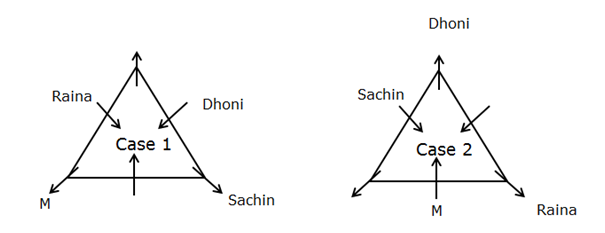I sits immediate left of the one who like Sachin.

Q likes Kholi and sits second to right of the one who like Yuvi.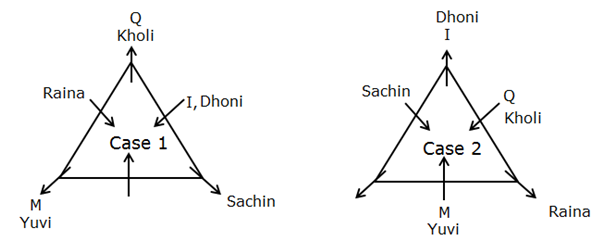L and J are not immediate neighbours.

S doesn’t like Sachin.

J doesn’t faces opposite to the centre.

The one who like Rohit sits second to the right of L.

From this above statement, Rohit sits immediate right of L in Case 1. So this Case is eliminated and Case 2 will be final arrangement.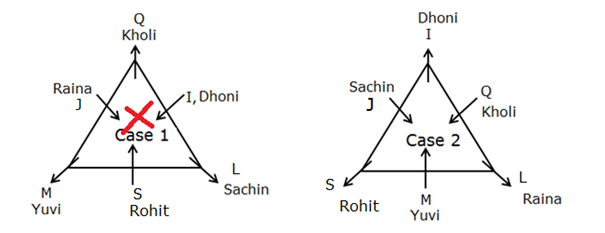6    21     X

7    11    25

For 2nd Row

• An odd number is followed by another odd number (but not a perfect square) so, 7+11 =18
• The row is 18 25 even number is followed by an odd (not a prime number) number 25-18 = 7 is the resultant of second row. Then we know resultant of two rows is 127, now we conclude that resultant of first row is 120.

For 1st Row,

• An Even number is followed by an odd number so = 21-6 = 15
• The row is 15 X , Odd number is followed by X we get the resultant of 120, we multiply 15 *8 then only we get 120. So 8 is the Answer.

3   6    9

4      7    27

For 1st Row

• An odd number is followed by an even number (not a cube number) 3*6 = 18
• The row is 18 9 , an even number is followed by an odd (not a prime number) number , 18-9 = 9(resultant of first row).

For 2nd Row

• An even number is followed by an odd number (prime number) then 7+4 = 11
• The row is 11   27 an odd number is followed by a perfect cube, then  27 -11 = 16( resultant of second row)
• Now sum of both the resultant is 25

4     7    4

6     7     X

• An even number is followed by an odd number (prime number) 4+ 7 =11
• The row is 11 4, an odd number is followed by an even number (not a cube number) 11 *4 = 44(resultant of first row)

X is double the value of resultant of  the first row , So X is 88.

6    7   88

• An even number is followed by an odd number (but not a perfect square) 6 + 7 = 13
• The row is 13  88, an odd number is followed by an even number, 13*88 = 1144

24  31   64

• An even number is followed by an odd 33+21= 55
• The row is 55 64 , an odd number is followed by a perfect cube 64-55 = 9

6   17   4

7    7   11

For 1st Row

• An even number is followed by an odd number(prime number) 6+17 = 23
• The Row is 23 4 an odd number is followed by an even number (not a cube number) then , 23*4 = 92(resultant of first Row)

For 2nd Row

• An odd number is followed by another odd number (but not a perfect square) 7 + 7 =14
• The Row is 14 11 an even number is followed by an odd number (prime number) then 14 +11 = 25(resultant of second Row)
• Difference of resultant of first row and second row = 92-25 = 67

Directions (9-10) :

From statement 1 we can conclude U is the cousin of W.

• S is Mother of U.
• S married V who is son-in law of P.
• T is daughter in law of P who married Q.
• R is father of W and married T.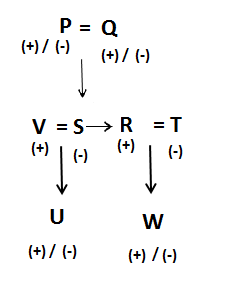From statement 2 we cannot conclude the gender of U hence we cannot get the relation.

• T is Son of U who has only one daughter.
• T married P.
• V is sister-in-law of P.
• V married S and has a daughter of W.
• S is son –in –law of Q who married U.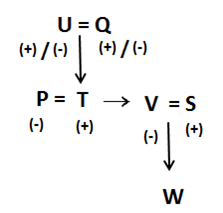Hence, statement I alone is sufficient to answer the question.

For Statement I we get the below diagram and we can easily find the distance between X and Y from the given data.

M walks 8m in north from point X , then he take right turn and walk 9mto reach point  S and then he take left turn and stop at point P after walking 10m. B walks 18m in north direction from point Y which is 35m east from point X and the he turn left and walk 10m and then turn right and walk 5m to reach point A. Then he walks 5m in west direction and then walks 5m in south direction and stop at point Q.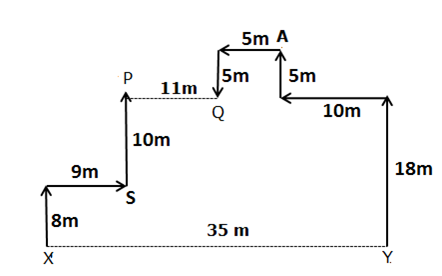For Statement II we get the below diagram and we can easily find the distance between X and Y from the given data.

M walks 5m in north direction from point X and take right and walks 5m, then walks 10m in south direction and stop at point P. N walks 5m in south direction and turn right and walk 20m to reach point D and then he walks 5m in north direction and stop at point Q. Point X is exactly between point Q and Y.